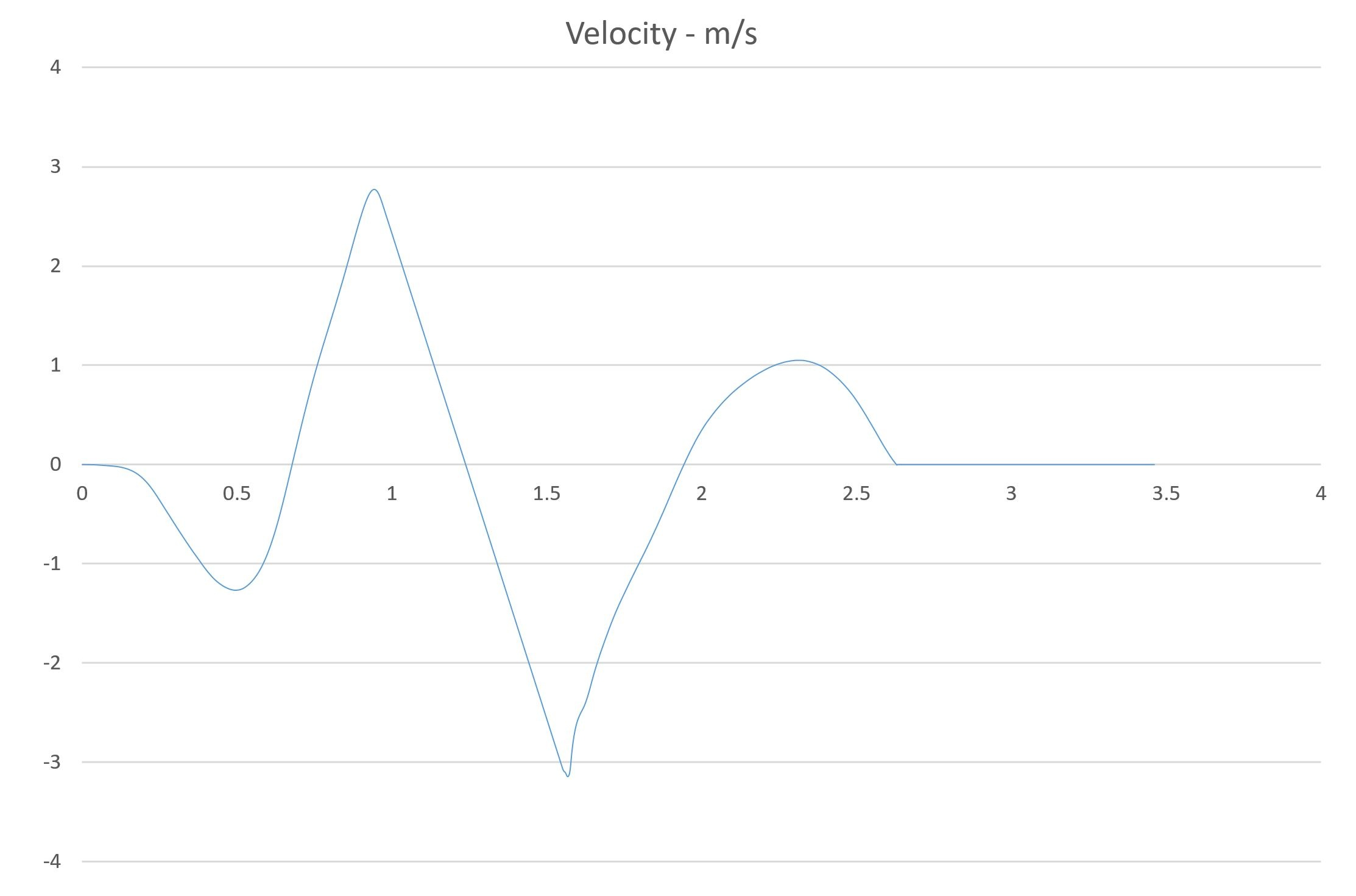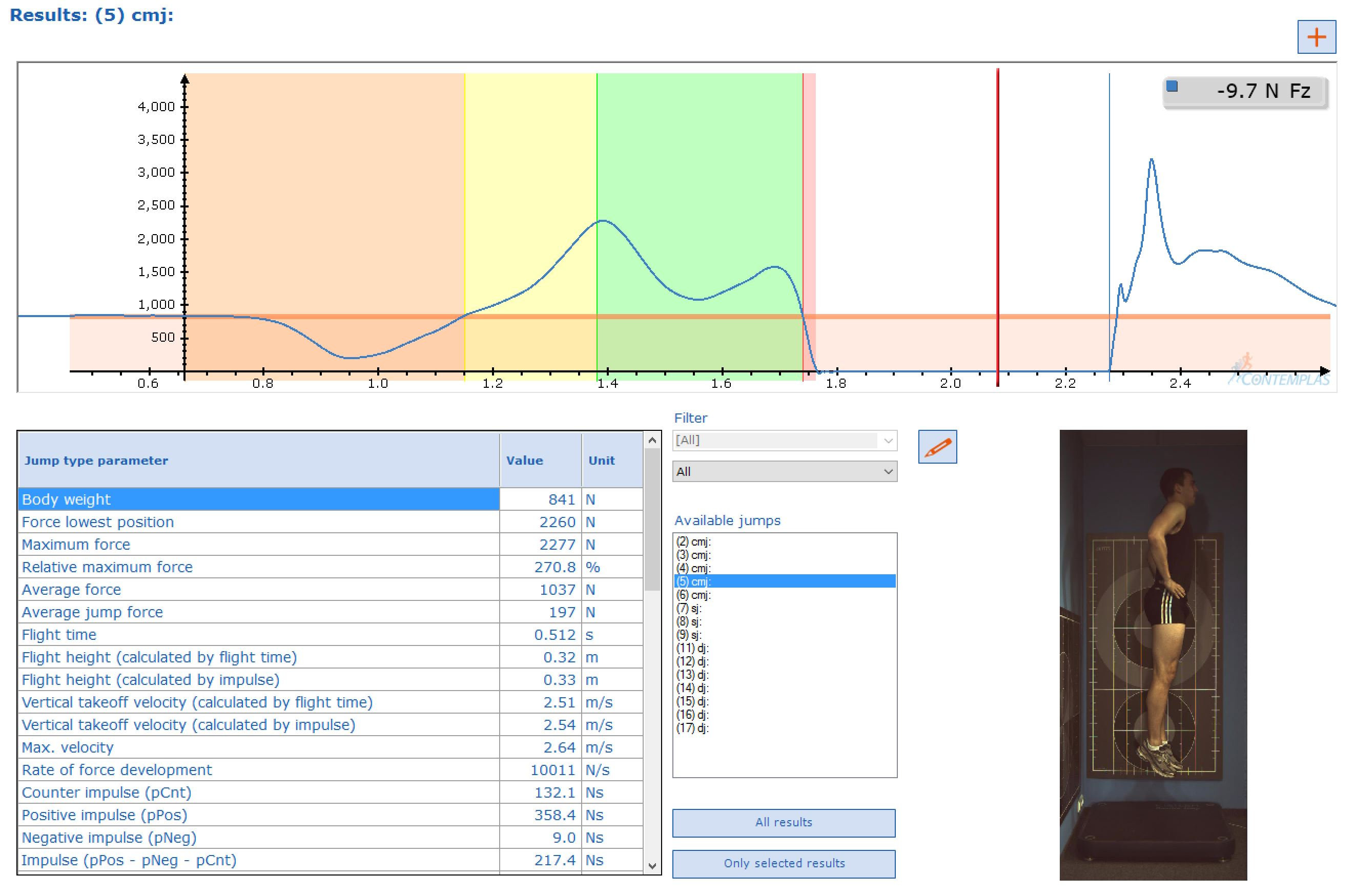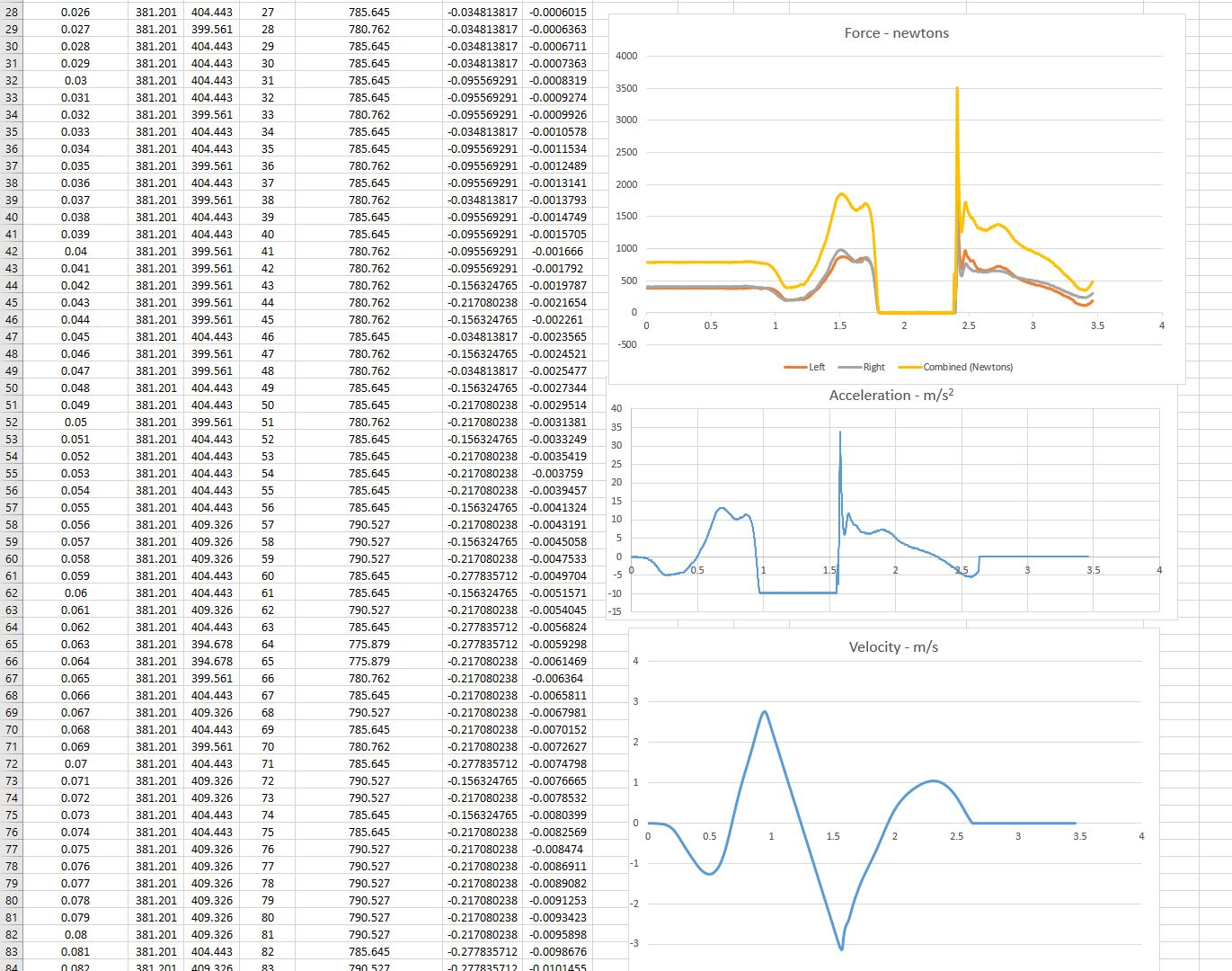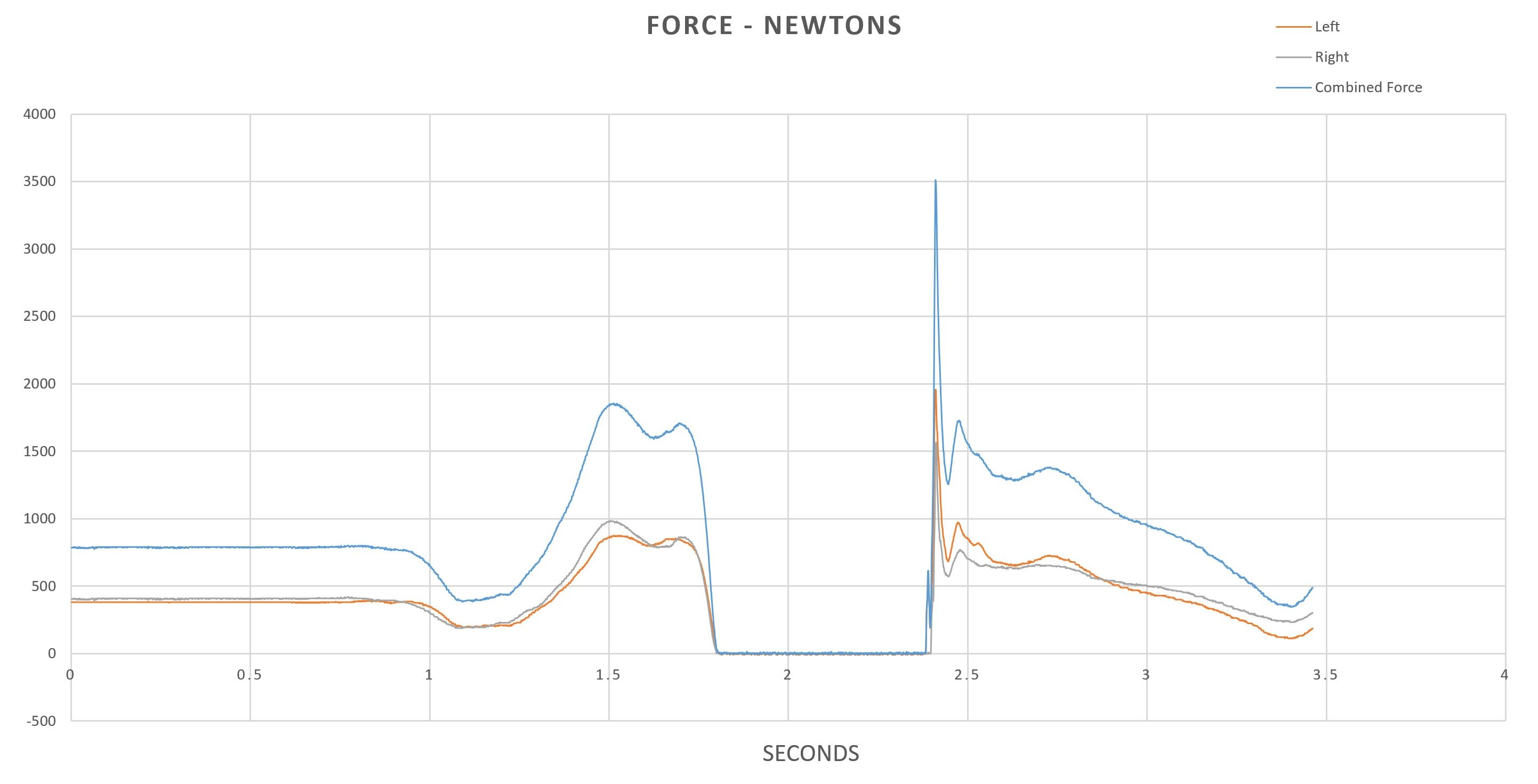# In Motion

After discussing jump measurement in general in a previous post, we thought it would be fitting to dive in and learn a bit more about what data we can obtain, and what we need to do to obtain it.  Dr. Jason Lake gives us some of his insights on CMJ measurement, and how these data can be managed and interpretted to provide value for athletes.

#### As you mentioned in our last conversation, the CMJ is an interesting movement.  What kind of data can we collect from the CMJ with force plates that we can’t get with other jump measurement systems/methods?

Well for a start, we can measure the force(s) applied to the centre of mass of the athlete or athlete/external load (typically a barbell) system rather than relying on a kinematics-based estimate. This normally involves measuring the displacement of a barbell, deriving acceleration by double differentiating displacement before applying Newton’s second law of motion by multiplying the mass of the barbell or barbell and lifter system to obtain force. While this sort of kinematics-based estimate of force tends to be pretty robust, the velocity and, as a consequence, power, tend to demonstrate poor agreement with velocity and power data obtained from force recorded from a force plate.

If we want to obtain power from our force data, we have to first calculate velocity. It is worth reiterating that data obtained from force recorded from a force platform refers to the force applied to the centre of mass and so velocity data reflects the velocity of the centre of mass. The first thing we need to do is subtract system weight from our force data, which yields what is often referred to as ‘net force’; dividing the result by system mass will then yield the acceleration of the centre of mass; integrating this with respect to time yields the velocity of the centre of mass. Displacement of the centre of mass can then be calculated by integrating our velocity data with respect to time. For those interested in the work performed during a movement, this can be calculated by multiplying force by displacement, although it is often better to calculate the area under the force displacement curve. Many skip this stage to get straight to power, which can be obtained by multiplying force by velocity.Researchers and practitioners are often interested in the impulse that’s applied to the centre of mass. This can be very useful, and some argue more so than power, because it underpins momentum. Impulse can be obtained by integrating net force with respect to time. Velocity-time data can be used to identify phases of interest and the impulse applied can then be identified by summing successively impulse across the phase of interest. If the impulse applied during the propulsion phase of countermovement jumping is obtained – typically from the post-countermovement transition from negative to positive velocity until take-off – it can then be divided by mass to provide the velocity of the centre of mass at that point. In this case this represents take-off and so velocity is take-off velocity. From this jump height can be calculated using the equations of uniform motion.While there are many more variables that are often of interest, and while there is a little more involved in calculating these variables and identifying phases accurately, this provides a pretty good start point.

#### Building off of that, I want to talk a bit more about velocities and accelerations derived from CMJ measurements—what is the best approach for calculating these variables and why are they important for monitoring and evaluating athletes?

I’ve already described how acceleration and velocity of the centre of mass can be obtained from force data recorded from a force plate (see above). This process can also seem quite daunting and this may explain the prevalence of measures, like peak force, that often tell us very little. This is where decent analysis software, propriety or custom, comes in. For example, for relatively simple calculations I’m still a big fan of Excel, which can help calm concerns because it provides a familiar interface. Combine the typical spreadsheet with a user-friendly interface and you can tackle an awful lot both effectively and efficiently. This may still seem daunting, but I’m more than happy to try and help point people in the right direction with this sort of thing.For those interested in taking this sort of thing a stage further, Matlab and LabVIEW tend to be the way to go. I have no experience with Matlab, and while my self-taught LabVIEW skills are probably quite clumsy, LabVIEW provides far more processing power than Excel. Again, where possible, I’m happy to try and help with this sort of thing. The key however is that certain guidelines need to be followed when collecting jump data. These include ensuring the athlete stands still for at least 1 s before they begin, providing clear and consistent instructions about what you want them to do, and not stepping off of the force platform until it has finished recording. These are pretty straight forward, but aren’t always implemented and make processing so much easier.

To answer the question though, velocity is key because of what it represents: How fast that athlete can move within the constraints of the test. This is important because it can be argued that it is velocity, and how quickly and efficiently one can change velocity with respect to time (acceleration), that is key to sports performance. Feeding back to an earlier question, and because athlete mass remains constant during the sorts of tests we’re talking about, any change in velocity -- more specifically any change in momentum – is underpinned by the impulse they can apply; remembering that impulse is the product of how much force (additional to their weight) they can apply during the time they have available.

#### This data can be intimidating to coaches, and we often get asked which values are most important to look at.  Jump height aside, what values do you think are most interesting to look at, and are most relevant to apply to the evaluation of athletes’ training regimen.I may buck the trend here, but I always try to keep an open mind. For example, I’m not a big fan of peak force or rate of force development. That may sound like sacrilege to many, and I’m also perfectly aware that lots of excellent sports scientists and practitioners have used these parameters effectively. It can of course also be argued that we should avoid ‘only use this available’ or ‘only use that variable’ dialogue as it’s about as useful as the exercise equivalents. However, I do think that we tend to overcomplicate things. With that in mind, my ‘go to’ parameter is impulse. I described this above, but it bears repeating: Impulse is the product of how much force (additional to body [and external load] weight) the athlete can apply during the time they have available. This provides us with a measure to quantify how hard the athlete can push in addition to providing a measure of how long they require to do this. We can also then identify their impulse at sports specific time points to establish performance potential. All of this is important because we can then also divide these impulse data by the mass of the athlete (plus external load if used) to calculate their velocity at this time point.

So from this simple measure we have the following:
i. How much force (additional to body [and external load] weight) they can apply during a particular test
ii. How long they take to apply this force, or…
iii. How much force they can generate at given time points specific to their sport
iv. Their velocity at the time points of interest

If you would like to get in touch with Dr. Lake, you can find him on twitter @DrJLake

Curious about force plates?  Reach out to our friends at Hawkin Dynamics!

Topics: How-To Posts and Guides